# Test: ER-Model- 2

## 20 Questions MCQ Test RRB JE for Computer Science Engineering | Test: ER-Model- 2

Description
Attempt Test: ER-Model- 2 | 20 questions in 60 minutes | Mock test for Computer Science Engineering (CSE) preparation | Free important questions MCQ to study RRB JE for Computer Science Engineering for Computer Science Engineering (CSE) Exam | Download free PDF with solutions
QUESTION: 1

### Consider the following relational schema. Students(rollno: integer, sname: string) Courses(courseno: integer, cname: string) Registration(rollno: integer, courseno: integer, percent: real) Q. Which of the following queries are equivalent to this query in English? "Find the distinct names of all students who score more than 90% in the course numbered 107"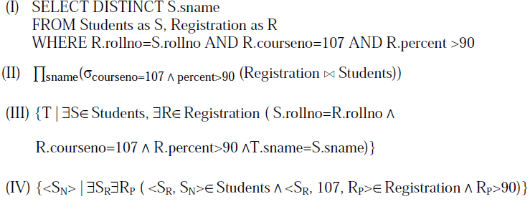Solution:

Option A:
This is a SQL query expression. It first perform a cross product of Students and Registration, then WHERE clause only keeps those rows in the cross product set where the student is registered for course no 107, and percentage is > 90. Then select distinct statement gives the distinct names of those students as the result set.

Option B:
This is a relational algebra expression. It first perform a NATURAL JOIN of Students and Registration (NATURAL JOIN implicitly joins on the basis of common attribute, which here is rollno ), then the select operation( sigma) keeps only those rows where the student is registered for courseno 107, and percentage is > 90. And then the projection operation (pi) projects only distinct student names from the set.
Note: Projection operation (pi) always gives the distinct result.

Option C:
This is a Tuple Relational Calculus (TRC) language expression, It is not a procedural language (i.e. it only tells “what to do”, not “how to do”). It just represents a declarative mathematical expression.
Here T is a Tuple variable.
From left to right, it can be read like this, “It is a set of tuples T, where, there exists a tuple S in Relation Students, and there exist a tuple R in relation Registration, such that S.rollno = R.rollno AND R.couseno = 107 AND R.percent > 90 AND T.sname = S.sname”. And the schema of this result is (sname), i.e. each tuple T will contain only student name, because only T.sname has been defined in the expression.
As TRC is a mathematical expression, hence it is expected to give only distinct result set.

Option D:
This is a Domain Relational Calculus (DRC) language expression. This is also not procedural. Here SN is a Domain Variable. It can be read from left to right like this “The set of domain variable SN, where, there exist a domain variable SR , and a domain variable Rp, such that, SN and SR domain variables is in relation Students and SR,107,RP is a domain variables set in relation Registration, AND RP > 90 “ Above, SN represents sname domain attribute in Students relation, SR represents rollno domain attribute in Students relation, and RP represents percentage domain attribute in Registration relation. The schema for the result set is (SN), i.e. only student name.

As DRC is a mathematical expression, hence it is expected to give only distinct result set.

QUESTION: 2

### Given the basic ER and relational models, which of the following is INCORRECT?

Solution:

The term ‘entity’ belongs to ER model and the term ‘relational table’ belongs to relational model. A and B both are true. ER model supports both multivalued and composite attributes See this for more details. (C) is false and (D) is true. In Relation model, an entry in relational table can can have exactly one value or a NULL.

QUESTION: 3

### Suppose (A, B) and (C,D) are two relation schemas. Let r1 and r2 be the corresponding relation instances. B is a foreign key that refers to C in r2. If data in r1 and r2 satisfy referential integrity constraints, which of the following is ALWAYS TRUE?

Solution:

B is a foreign key in r1 that refers to C in r2. r1 and r2 satisfy referential integrity constraints. So every value that exists in column B of r1 must also exist in column C of r2.

QUESTION: 4

Consider the following relations A, B, C. How many tuples does the result of the following relational algebra expression contain? Assume that the schema of A U B is the same as that of A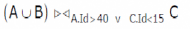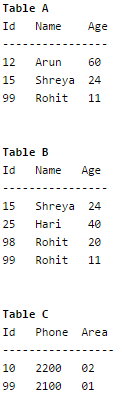Solution:

Result of AUB will be following table

Id   Name    Age
----------------
12   Arun    60
15   Shreya  24
99   Rohit   11
25   Hari    40
98   Rohit   20

The result of given relational algebra expression will be

Id   Name    Age  Id   Phone Area
---------------------------------
12   Arun    60   10   2200   02
15   Shreya  24   10   2200   02
99   Rohit   11   10   2200   02
25   Hari    40   10   2200   02
98   Rohit   20   10   2200   02
99   Rohit   11   99   2100   01
98   Rohit   20   99   2100   01

QUESTION: 5

Consider a relational table r with sufficient number of records, having attributes A1, A2,…, An and let 1 <= p <= n. Two queries Q1 and Q2 are given below.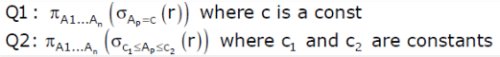Q. The database can be configured to do ordered indexing on Ap or hashing on Ap. Which of the following statements is TRUE?

Solution:

If record are accessed for a particular value from table, hashing will do better. If records are accessed in a range of values, ordered indexing will perform better.

QUESTION: 6

Which of the following tuple relational calculus expression(s) is/are equivalent to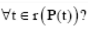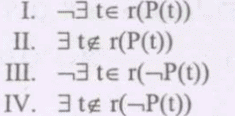Solution:

Some transformation rules for tuple relational calculus are :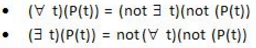Thus, using the above rules option (C) is correct.

QUESTION: 7

Let R and S be two relations with the following schema R (P,Q,R1,R2,R3) S (P,Q,S1,S2) Where {P, Q} is the key for both schemas. Which of the following queries are equivalent?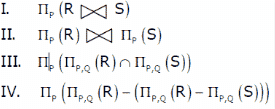Solution:
QUESTION: 8

Consider the following ER diagram.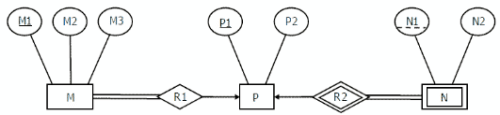The minimum number of tables needed to represent M, N, P, R1, R2 is

Solution:

Answer is B, i.e, 3 minimum tables. M, P are strong entities hence they must be represented by separate tables. Many-to-one and one-to-many relationship sets that are total on the many-side can be represented by adding an extra attribute to the “many” side, containing the primary key of the “one” side. ( This way no extra table will be needed for Relationship sets ) M table is modified to include primary key of P side(i.e. P1). N is weak entity, and is modified to include primary key of P (i.e, P1). Therefore there would be minimum of 3 tables with schema given below :
M (M1, M2, M3, P1)
P (P1, P2)
N (P1, N1, N2)

Note: This modification of a table in the case of one-many or many-one to include relationship set at the many side works well, but only in the case when the relationship set doesn't have its own attributes. If the relationship set has its own attribute then we need to make a separate table for the relationship set also.

QUESTION: 9

Consider the data given in above question. Which of the following is a correct attribute set for one of the tables for the correct answer to the above question?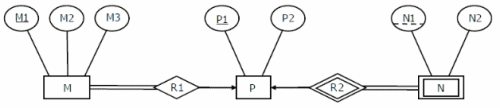Solution:

We get 3 tables. M: {M1, M2, M3, P1} P: {P1, P2} N: {P1, N1, N2} The only attribute set that matches the given table sets is A. Therefore, option A

QUESTION: 10

Information about a collection of students is given by the relation studinfo(studId, name, sex). The relation enroll(studId, courseId) gives which student has enrolled for (or taken) that course(s). Assume that every course is taken by at least one male and at least one female student. What does the following relational algebra expression represent?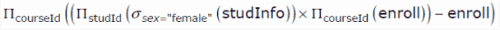Solution:

The expression given in question does following steps in sequence.
a) Select studids of all female students and selects all courseids of all courses.
b) Then the query does a Cartesian Product of the above select two columns from different tables.
c) Finally it subtracts enroll table from the result of above step (b). This will remove all the (studid, courseid) pairs which are present in enroll table. If all female students have registered in a courses, then this course will not be there in the subtracted result.
So the complete expression returns courses in which a proper subset of female students are enrolled.

QUESTION: 11

Consider the relation employee(name, sex, supervisorName) with name as the key. supervisorName gives the name of the supervisor of the employee under consideration. What does the following Tuple Relational Calculus query produce?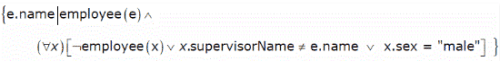Solution:

OR (∨) is commutative and associative, therefore i can rewrite given query as: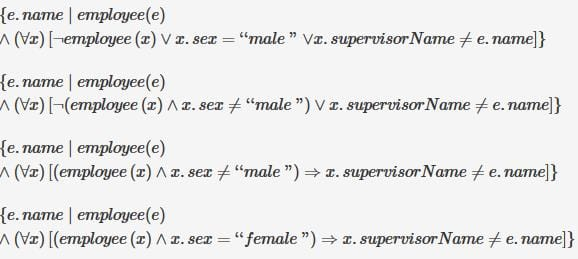It is clear now they are saying something about female employees, This query does not say anything about male employees. Therefore Option A and B are out of consideration.

This query retrieves those e.name who satisfies this condition: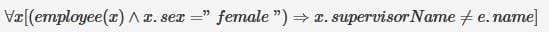Means retrieves those e.name, who is not a supervisor of any female employees.
i.e it retrieves name of employees with no female subordinate.
(here "immediate" is obvious, as we are checking first level supervisor.)
Hence, option C.

QUESTION: 12

Consider a join (relation algebra) between relations r(R)and s(S) using the nested loop method. There are 3 buffers each of size equal to disk block size, out of which one buffer is reserved for intermediate results. Assuming size(r(R)) < size(s(S)), the join will have fewer number of disk block accesses if

Solution:

Nested loop join is one of the methods to implement database in memory. A nested loop join is an  algorithm that joins two sets by using two nested loops.

According to nested join,given relation R and S   For each tuple r in R do For each tuple s in S do If r and s satisfy the join condition Then output the tuple <r,s> Cost estimations for the above loop: – b(R) and  b(S) number of blocks in R and in S – Each block of outer relation is read once – Inner relation is read once for each block of outer relation Summing up : IO= b(R)+b(R)*b(S) total  IO operations Lets assume |R|>|S|  i.e b(R) =10  and b(s) =3 Now,   if R is outer relation then, IO= 10+10*3=40 if S is outer relation then IO=3+10*3=33 As it can be observed , that total IO is lesser if the value of outer variable is less and as it is already given that |R|<|S|.Therefore,  Relation r(R) should be in the outer loop to have fewer number of disk block accesses.

QUESTION: 13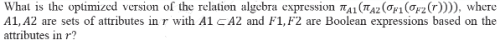Solution:

The Relational Algebra expression in the question above, does 4 operations, step by step ( innermost braces first ) .

1. Select those tuples from relation r which satisfies expression/condition F1, say the result of this operation is set A.
2. Select those tuples from set A which satisfies expression/condition F2, say the result of this operation is set B.
3. Select attrributes set A2 from set B, say the result of this operation is set C.
4. Select attrributes set A1 from set C, say the result is set D which is the final result.

Now to optimize this expression, we can combine operations/steps 1 and 2 by AND operator between F1 and F2 condition, like F1 ^ F2, and instead of selecting first attribute set A2, we can directly select attribute set A1 from the result of the combined operation, which is represented by expression in Option A .

QUESTION: 14

Consider the relational schema given below, where eId of the relation dependent is a foreign key referring to empId of the relation employee. Assume that every employee has at least one associated dependent in the dependent relation.

employee (empId, empName, empAge) dependent(depId, eId, depName, depAge)

Consider the following relational algebra query: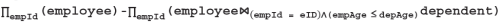Q. The above query evaluates to the set of empIds of employees whose age is greater than that of

Solution:

The below subquery after the subtraction sign produces id's of those employees who have at least one dependent with age greater than or equal the employee's age.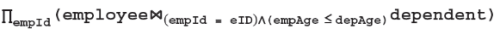When the result of above subquery is subtracted from all employees, we get the employees whose age is greater than all dependents.

QUESTION: 15

Let E1 and E2 be two entities in an E/R diagram with simple single-valued attributes. R1 and R2 are two relationships between E1 and E2, where R1 is one-to-many and R2 is many-to-many. R1 and R2 do not have any attributes of their own. What is the minimum number of tables required to represent this situation in the relational model?

Solution:

The answer is B, i.e minimum 3 tables. Strong entities E1 and E2 are represented as separate tables. In addition to that many-to-many relationships(R2) must be converted as seperate table by having primary keys of E1 and E2 as foreign keys. One-to-many relaionship (R1) must be transferred to 'many' side table(i.e. E2) by having primary key of one side(E1) as foreign key( this way we need not to make a seperate table for R1). Let relation schema be E1(a1,a2) and E2( b1,b2). Relation E1( a1 is the key)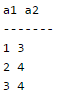Relation E2( b1 is the key, a1 is the foreign key, hence R1(one-many) relationship set satisfy here)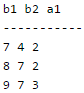Relation R2 ( {a1, b1} combined is the key here , representing many-many relationship R2)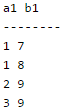Hence we will have minimum of 3 tables.

QUESTION: 16

In a schema with attributes A, B, C, D and E following set of functional dependencies are given

A → B A → C CD → E B → D E → A

Q. Which of the following functional dependencies is NOT implied by the above set?

Solution:

Apply membership test for all the given Functional Dependencies.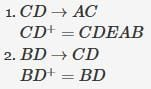i.e. BD cannot derive CD

and hence is not implied.

Similarly do for rest two.

QUESTION: 17

A database of research articles in a journal uses the following schema.

(VOLUME, NUMBER, STARTPGE, ENDPAGE, TITLE, YEAR, PRICE)
The primary key is (VOLUME, NUMBER, STARTPAGE, ENDPAGE) and the following functional dependencies exist in the schema.

(VOLUME, NUMBER, STARTPAGE, ENDPAGE) -> TITLE (VOLUME, NUMBER) -> YEAR (VOLUME, NUMBER, STARTPAGE, ENDPAGE) -> PRICE
The database is redesigned to use the following schemas.

(VOLUME, NUMBER, STARTPAGE, ENDPAGE, TITLE, PRICE) (VOLUME, NUMBER, YEAR)

Q. Which is the weakest normal form that the new database satisfies, but the old one does not?

Solution:

Volume, Number -> Year is partial dependency. So it does not follow 2NF. But decomposed relation follows.

QUESTION: 18

Which of the following relational query languages have the same expressive power?

1. Relational algebra
2. Tuple relational calculus restricted to safe expressions
3. Domain relational calculus restricted to safe expressions
Solution:

Relational algebra is a procedural query language where we input - relations and it yields relations as output. It provides method to get the result. It is performed recursively on a relation and the in between results are relations(output). Basic set of operations for the relational model. Relational calculus is a non - procedural query language. It provides the query to get result. Higher level declarative language for specifying relational queries. Tupple Relational Calculus operates on each tupple. Domain Relational Calculus operates on each column or attribute. Safe expression means fixed no. of tupple or column or attribute as a result But all of them has same expressive power. Just different ways to do so.

QUESTION: 19

A Relation R with FD set {A->BC, B->A, A->C, A->D, D->A}. How many candidate keys will be there in R?

Solution:

Simple candidate key means single attributed key. As (A)+ = {A, B, C, D}, (B)+ = {B,A, C, D}, (C)+ = {C} and (D)+ = {D, A, B, C}. So, A, B and D are candidate keys which are simple as well. So, correct option is 3.

QUESTION: 20

What is the min and max number of tables required to convert an ER diagram with 2 entities and 1 relationship between them with partial participation constraints of both entities?

Solution:

Maximum number of tables required is 3 in case of many to many relationships between entities. Minimum number of tables is 1 in case of unary relationship and total participation of atleast one entity. But in case of partial participation of both entities, minimum number of tables required is 2.Use Code STAYHOME200 and get INR 200 additional OFF Use Coupon Code